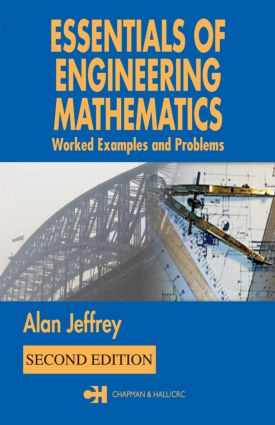Essentials Engineering Mathematics

2nd Edition

Chapman and Hall/CRC

896 pages | 233 B/W Illus.

Purchasing Options:\$ = USD
Paperback: 9781584884897
pub: 2004-08-12
SAVE ~\$20.00
\$100.00
\$80.00
x
Hardback: 9781138442573
pub: 2017-07-27
SAVE ~\$41.00
\$205.00
\$164.00
x
eBook (VitalSource) : 9780429178191
pub: 2004-08-12
from \$49.48

FREE Standard Shipping!

Description

First published in 1992, Essentials of Engineering Mathematics is a widely popular reference ideal for self-study, review, and fast answers to specific questions. While retaining the style and content that made the first edition so successful, the second edition provides even more examples, new material, and most importantly, an introduction to using two of the most prevalent software packages in engineering: Maple and MATLAB. Specifically, this edition includes:

• Introductory accounts of Maple and MATLAB that offer a quick start to using symbolic software to perform calculations, explore the properties of functions and mathematical operations, and generate graphical output

• New problems involving the mean value theorem for derivatives

• Extension of the account of stationary points of functions of two variables

• The concept of the direction field of a first-order differential equation

• Introduction to the delta function and its use with the Laplace transform

The author includes all of the topics typically covered in first-year undergraduate engineering mathematics courses, organized into short, easily digestible sections that make it easy to find any subject of interest. Concise, right-to-the-point exposition, a wealth of examples, and extensive problem sets at the end each chapter--with answers at the end of the book--combine to make Essentials of Engineering Mathematics, Second Edition ideal as a supplemental textbook, for self-study, and as a quick guide to fundamental concepts and techniques.

• Reviews

"The book is intended for first year engineering students and presumably this choice of subjects is a reflection of the course on which the author lectured. The book fulfills this purpose in a very satisfactory manner and can be warmly recommended for this purpose. The explanations are good and there is an adequacy of worked examples. …A welcome feature is that indefinite integrals mentioned in the text all have included an arbitrary constant. …Much of the book would be found useful for those in the last years at school."

-Zentralblatt MATH

"Each of the short sections covers the amount of material one would hope to get through in a lecture or two, typically giving a short introduction to the relevant theory and several worked examples. …Jeffrey's book could be easily adopted as a course text, and the sections can be divided naturally into groups for shorter modules."

-Times Higher Education Supplement

ead> Concise explanations and a wealth of examples form an ideal study guide and reference

Real numbers, inequalities and intervals

Function, domain and range

Basic coordinate geometry

Polar coordinates

Mathematical induction

Binomial theorem

Combination of functions

Symmetry in functions and graphs

Inverse functions

Complex numbers; real and imaginary forms

Geometry of complex analysis

Modulus-argument form of a complex number

Roots of complex numbers

Limits

One-sided limits

Derivatives

Leibniz's formula

Differentials

Differentiation of inverse trigonometric functions

Implicit differentiation

Parametrically defined curves and parametric differentiation

The exponential function

The logarithmic function

Hyperbolic functions

Inverse hyperbolic functions

Properties and applications of differentialability

Functions of two variables

Limits and continuity of functions of two real variables

Partial differentiation

The total differential

The chain rule

Change of variable in partial differentiation

Antidifferentiation (integration)

Integration by substitution

Some useful standard forms

Integration by parts

Partial fractions and integration of rational functions

The definite integral

The fundamental theorem of integral calculus and the evaluation of definite integrals

Improper integrals

Numerical integration

Geometrical applications of definite integrals

Centre of mass of a plane lamina

Applications of integration to he hydrostatic pressure on a plate

Moments of inertia

Sequences

Infinite numerical series

Power series

Taylor and Maclaurin series

Taylor's theorem for functions of two variable: stationary points and their identification

Fourier series

Determinants

Matrices

Matrix multiplication

The inverse matrix

Solution of a system of linear equations: Gaussian elimination

The Gauss-Seidel iterative method

The algebraic eigenvalue problem

Scalars, vectors and vector addition

Vectors in component form

The straight line

The scalar product (dot product)

The plane

The vector product (cross product)

Applications of the vector product

Differentiation and integration of vectors

Dynamics of a particle and the motion of a particle in a plane

Scalar and vector fields and the gradient of a scalar function

Ordinary differential equations: order and degree, initial and boundary conditions

First order differential equations solvable by separation of variables

The method of isoclines and Euler's methods

Homogeneous and near homogeneous equations

Exact differential equations

The first order linear differential equation

The Bernoulli equation

The structure of solutions of linear differential equations of any order

Determining the complementary function for constant coefficient equations

Determining particular integrals of constant coefficient equations

Differential equations describing oscillations

Simultaneous first order linear constant coefficient different equations

The Laplace transform and transform pairs

The Laplace transform of derivatives

The shift theorems and the Heaviside step function

Solution of initial value problems

The Delta function and its use in initial value problems with the Laplace transform

Enlarging the list of Laplace transform pairs

Symbolic Algebraic Manipulation by Computer Software